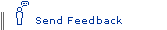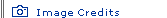Smashing Pictures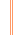The Elegant Universe homepage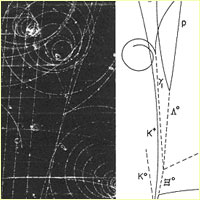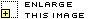This image shows the first Omega-minus particle to be discovered. An incoming K- meson collides with a proton in a liquid hydrogen bubble chamber producing the Omega-minus, a K+ meson, and a K0 meson. Neutral particles leave no tracks in the chamber and are denoted by dashed lines. The positions of the neutral particles are inferred from the visible decay products and by the use of the laws of conservation of energy and momentum. Discovery of the strange quark One of the most important observations in particle physics was the discovery in 1964 of the Omega-minus baryon at Brookhaven National Laboratory. In the two decades leading up to this point over 27 supposedly "fundamental" particles had been found. In 1961 Murray Gell-Mann and Yuvall Ne'eman independently noticed that these particles could be grouped by a symmetry called SU(3)—the special unitary group in three dimensions—into a new "periodic table" called "the Eightfold Way." In this scheme the particles with the same quantum numbers of spin and parity are collected into singlets, octets, and decuplets (groups of 1, 8, and 10). Within these groups, the particles differed in their mass, charge, and a funny quantum number called strangeness. In 1964 the only particle missing from the decuplet was the one that had three units of strangeness. Using the known baryons, this particle was predicted to have spin 3/2, charge -1, and a mass of roughly 1.7 GeV/c^2. The subsequent discovery of the Omega-minus with exactly these properties confirmed that there was an underlying symmetry that described matter. Further, the difference in masses of the particles broke the symmetry in a systematic and predictive manner. After the discovery of the Omega-minus, Gell-Mann and George Zweig proposed that this SU(3) symmetry was actually an artifact of the fact that the baryons were made of three "quarks"—the up, down, and strange quarks—which had charges +2/3, -1/3, and -1/3, respectively. Hence, the Omega-minus is a collection of three strange quarks. The quark model was born.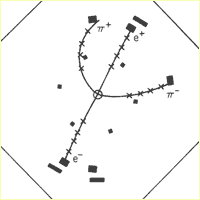Computer reconstruction of the decay of a Psi-prime meson into J/Psi, pi+, and pi- mesons. The shape of this event resembles the Greek letter Psi and was jokingly offered as proof that the particle knew its name. Discovery of the charm quark Prior to 1970, it was understood that the weak force could cause a strange quark (charge -1/3) to decay into an up quark (charge +2/3), a muon (charge -1), and a neutrino (charge 0). It was a puzzle, however, as to why the strange quark was never observed to decay into a down quark (charge -1/3) plus two neutrinos. Along with this puzzle was the observation that while physicists had discovered four leptons—electron, muon (a heavy version of the electron), electron neutrino, and muon neutrino—they had seen only three quarks—up, down, and strange. In 1970 Sheldon Glashow, John Iliopoulos, and Luciano Maiani proposed that if there was a fourth quark with charge +2/3, then a symmetry would forbid these "neutral current" decays. In 1974 physicists at both Brookhaven National Laboratory and the Stanford Linear Accelerator Center discovered a new meson called the J/Psi. This meson was seen as a peak in the annihilation of electron/anti-electron pairs at a mass of 3.1 GeV/c^2. The striking feature of this discovery was that the particle lived over 1,000 times longer than expected. This meant that it could not be just a composition of the up, down, and strange quarks but was composed of a charm/anti-charm quark pair. The discovery of the charm quark left no doubt that there was a deep symmetry that forced the number of leptons and quarks to be the same.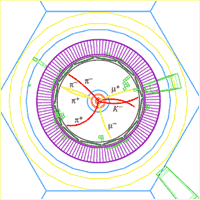Computer reconstruction of the decays of a B meson and an anti-B meson from the BABAR experiment at the Stanford Linear Accelerator Center. One B meson (gold tracks) decays into a J/Psi (which decays to mu+ and mu-) and K-short (which decays to pi+ and pi-). The other B meson (red tracks) decays into a K- and three pions. Discovery of the bottom quark When the tau lepton was discovered in 1975, the number of known leptons was increased to five (electron, muon, tau, electron neutrino, and muon neutrino). Immediately a search began for a third generation of quarks: the bottom (or beauty) quark and top (or truth) quark. In 1977 physicists discovered a new meson called the Upsilon at the Fermi National Accelerator Laboratory. This meson was immediately recognized as being composed of a bottom/anti-bottom quark pair. The bottom quark had charge -1/3 and a mass of roughly 5 GeV/c^2. We owe our existence to the fact that the universe does not have the same amount of matter and antimatter. Despite this observation, it was commonly believed that the laws of physics were subject to a symmetry known as CP, which states that if matter is exchanged with antimatter and space is inverted (think of a reflection in a mirror), then the laws of physics should not change. In 1964 James Cronin and Val Fitch discovered that this symmetry is violated by the weak force at 1 part in 1,000 in mesons that had strange quarks. In 1973 Makoto Kobayashi and Toshikide Maskawa showed that if there were six quarks, then this CP violation could be explained by mixing between the down, strange, and bottom quarks. The mixing leads to prediction of a large asymmetry in the decays of B mesons to J/Psi and K-short mesons. The observation of this asymmetry provided strong support for the Kobayashi-Maskawa hypothesis.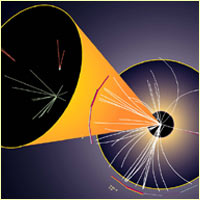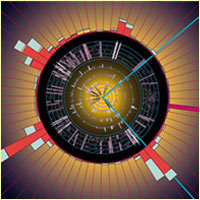The first image shows a pair of top quarks reconstructed in the Collider Detector at Fermilab (CDF). Each top quark decays to a W boson and a b quark. The pink tower in the wide view identifies a positron (an anti-electron) from one W decay; the inset shows displaced decays of two b particles (red tracks). The second image shows a pair of top quarks reconstructed in the DZero experiment at Fermilab. This end view shows the final decay products: two muons (turquoise), a neutrino (pink), and four jets of particles. The height of the boxes denotes the amount of energy deposited in the detector in each wedge. Discovery of the top quark After the discovery of the bottom quark in 1977, physicists expected to quickly find its partner called the top quark. But it required 18 years of dedicated work to identify the production of just 20 pairs of top quarks. In 1995 physicists identified the top quark by both the CDF and DZero experiments at Fermilab. Many physicists were amazed to find that the mass of the top quark was 175 GeV/c^2 (similar to a gold atom), which is about 35 times as massive as the bottom quark and about 35,000 times as massive as the up and down quarks. The standard model of particle physics predicts that early in the history of the universe, before the electroweak force split into the electromagnetic and weak forces, all fundamental matter particles (quarks and leptons) were exactly massless. When the forces split, a symmetry relating left- and right-handed particles called chirality was also broken, allowing particles to acquire masses. Remarkably, if we assume that all unknown numerical coefficients should be approximately equal to 1, then the top quark mass is predicted to be 175 GeV/c^2! Unfortunately, this would also predict that all other matter particles would have masses of around 175 GeV/c^2, which is in rather poor agreement with observation. One of the most profound mysteries of nature is why most of matter is so light compared to the top quark. As the top quark continues to be studied at Fermilab, a unique property of the decay of the top quark will play a vital role in understanding what physics could lie beyond the standard model. It turns out that the top quark decays before it has time to bind to another quark. This implies that measurements of the decays probe the actual properties of the quark itself, and are not washed out by additional interactions. A significant fraction of theoretical models that go beyond the standard model predict significant deviations of the measured rates of production, the angles measured between the decay products, and even what the decay products will be. The standard model predicts most of these quantities to high precision, and any deviation will be a sign of new physics.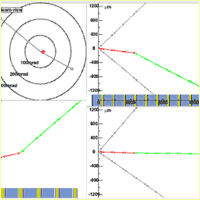A tau lepton appearing in a tau neutrino interaction as reconstructed from an emulsion (photographic) measurement. The tau lepton (red track) appears in the middle of the detector with two associated particles (most likely pions in gray). The tau travels a short distance and decays into an electron (green track) and two invisible neutrinos, which causes a kink in the track. Discovery of the tau neutrino The tau neutrino was the last matter particle of the standard model to be observed. After the discovery of the tau lepton in 1975, there was little doubt that the tau neutrino existed. Later measurements of the invisible decay of the Z boson led to an indirect measurement that there were exactly three light neutrinos. However, it was not until 2001 that a tau neutrino was directly detected for the first time by the DONUT experiment at Fermilab. The scale of the problem is best described by realizing that 20 trillion neutrinos pass through your living room every second, and yet it is likely that none will ever interact with anything. In order to "see" a tau neutrino, an experiment has to look for a tau lepton that appears out of nowhere but points back to the source of tau neutrinos.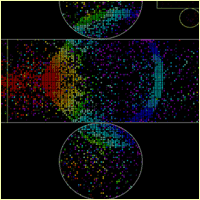One method of measuring neutrino oscillation is to compare the difference in the number of muon neutrinos produced by the KEK proton synchrotron accelerator in Tsukuba, Japan, and the number that reach the Super-Kamiokande detector 155 miles away. In this image a muon neutrino interacts inside the 1,000-ton water tank at KEK and creates a muon. The muon emits a ring of Cerenkov light as it slows down in the water. The color coding denotes the time of arrival of the light and is used to verify that the neutrino came from the direction of the accelerator. Neutrino mass and oscillation One of the great successes of astrophysics is the solar standard model. This model predicts with high accuracy how fusion of elements in the sun ultimately produces the light that we see. Electron neutrinos are emitted in the process of fusion. These neutrinos interact so weakly that only a handful of the billion billion that pass through a detector each day are seen. However, it was realized as early as 1967 that physicists were finding only half of the electron neutrinos predicted to be coming from the sun. The solution to the mystery of the disappearing neutrinos begins with the 1998 observation by the Super-Kamiokande collaboration in Japan that a large fraction of the muon neutrinos produced in the atmosphere were oscillating into tau neutrinos before they reached the Earth. In 2002 the Sudbury Neutrino Observatory in Ontario, Canada, confirmed that the total number of neutrinos coming from the sun is exactly consistent with the prediction of the solar standard model, but that only half of these are electron neutrinos. The combination of these observations leads to the conclusion that the rest of the electron neutrinos coming from the sun oscillate into either muon neutrinos or tau neutrinos. While neither of these experiments measure the actual neutrino masses, the fact that neutrinos mix overturns a 50-year-old assumption that the masses were exactly zero. Several ongoing experiments are attempting to understand the exact nature of neutrino mixing and to determine the neutrino masses. The discovery that neutrinos of different generations can turn into each other violates a symmetry known as lepton family number conservation. In all known particle physics experiments performed so far, the total number of leptons of a given type is always the same. Therefore, if a muon (muon-L = 1) decays into an electron (electron-L= 1), then there is always a muon neutrino (muon-L = 1), and an electron anti-neutrino (electron-L = -1) as well. If a muon neutrino can become an electron anti-neutrino, then the decay of a muon to an electron plus a photon should be possible. This has never been seen, however, because the probability of such a decay occurring is less than one part in a million trillion trillion trillion trillion. It is unlikely that this has ever occurred in our galaxy.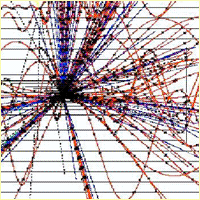The first image is a reconstruction of a W boson produced in a proton/anti-proton collision. The W boson quickly decays into a tau lepton and tau anti-neutrino. The pencil-like jet in the center is the tau lepton, the neutrino is inferred from energy conservation, and the curved tracks are the remnants of the proton and anti-proton. The second image is a reconstruction of a proton/anti-proton collision that produced a Z boson. The Z boson decayed to an electron and a positron (blue dotted tracks). The other tracks are the remnants of the proton and anti-proton. Electroweak symmetry The electromagnetic force between charges (responsible for both chemical binding and the emission of light) is carried by a massless photon. The weak force is responsible for both the fusion of hydrogen in the sun into deuterium and the decay of a neutron into a proton, electron, and anti-neutrino. In the 1960s Sheldon Glashow, Abdus Salam, and Steven Weinberg realized that a single theory of electroweak interactions could describe these two forces. This theory showed that the weak force was weak because it occurred by the exchange of very massive W and Z bosons, which have a very short range. The W boson changes one particle into another with a different charge—for example, an electron (charge -1) into an electron neutrino (charge 0), or a strange quark (charge -1/3) into an up quark (charge +2/3). The Z boson is similar to the photon in that it does not change particles into each other. However, the Z boson can interact with neutrinos, whereas the massless photon only interacts with charged particles. In 1967 Steven Weinberg showed that there was a fixed relationship between masses of the W and Z bosons. In 1983 the W and Z bosons were found by the UA1 collaboration at the Super Proton Synchrotron at CERN with exactly the masses they were predicted to have. Today many of the predictions of the electroweak theory have been tested to high precision. However, there is one additional neutral particle called the Higgs boson that is predicted by the theory, but has not been found. There are no exact predictions of the mass of the Higgs boson, but indirect measurements suggest that this particle should be seen in the near future. A tantalizing prospect is that the same indirect measurements suggest that the theory may not be complete. Hence, the search for the Higgs boson may soon completely change our understanding of the forces of nature.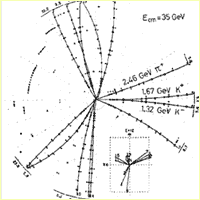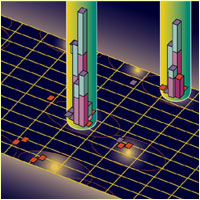In the first image, the production of three jets (or isolated groupings) of particles indicates the discovery of the gluon. Two jets are the remnants of quarks, and one is from a gluon. As the second image indicates, modern detectors can see gluons by the production of three jets. The height of the boxes indicates the amount of energy deposited in the detector, and the colors indicate how far into the detector the energy was deposited. Production of the gluon The quark model explained why mesons are made of quark/anti-quark pairs, and baryons are made of three quarks or three anti-quarks. This appears to violate the Pauli exclusion principle, which forbids putting even two identical quarks in the same state. And yet the Omega-minus baryon (composed of three strange quarks) was discovered in 1964. The solution to this puzzle, proposed by Oscar Greenberg in 1964, is that the quarks each carry a "color" charge (red, blue, or green). Hence, baryons are composed of one red quark, one blue quark, and one green quark, and mesons are composed of one red quark and one anti-red anti-quark (or green/anti-green, or blue/anti-blue). All observable particles must have no net color (red + green + blue = 0). The mathematics that describes how the colors can combine is the same SU(3)—the special unitary group in three dimensions—that led to the discovery of quarks. Unlike the approximate symmetry between the quarks, however, this symmetry is exact. This theory predicted that the quarks are bound together by a strong force that is carried by a new massless particle called the gluon. The gluon connects one color to another in eight possible ways, but since it carries color charge itself, it is never seen in isolation. In 1979 scientists at the PETRA storage ring at the DESY laboratory in Hamburg, Germany observed three jets of particles for the first time. Two of the jets were due to the hadronization (the process of becoming color neutral) of quarks, and one was from a gluon.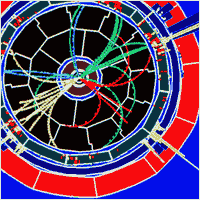This image shows a candidate for the associated production of a Higgs boson and Z boson. The candidate Higgs decays to a bottom quark and anti-bottom quark, which in turn decay to the jets denoted by the green and yellow tracks. The Z boson also decays to two jets denoted by the red and blue tracks. Search for the Higgs boson The electroweak symmetry that describes both the electromagnetic and weak forces is not exact at low energy. This symmetry is broken by the Higgs mechanism, which predicts the existence of a new particle with no spin called the Higgs boson. The interaction between Higgs bosons and quarks or leptons provides the mass of these fundamental matter particles. Despite 30 years of searching, the Higgs boson has remained elusive. Indirect evidence suggests that discovery of the Higgs boson should be within reach of experiments that will occur over the next decade at Fermilab and the CERN Large Hadron Collider. The difficulty in finding the Higgs boson has created a great deal of excitement in particle physics. Whether or not the Higgs exists, the standard model is now known to be incomplete. If the Higgs is found, then a calculation of the Higgs mass implies that the standard model breaks down at energies just higher than the mass of the Higgs itself. By studying the properties of the Higgs boson it is hoped that clues can be found to what lies beyond. An even more exciting possibility is that the particle does not exist. In this case there would be completely new types of matter that have never been seen before. The structure of space and time itself may even be different than expected.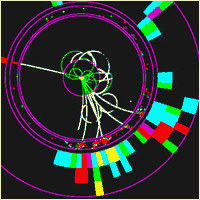This is a simulation of the production and decay of supersymmetric particles in a proposed linear collider detector. The straight line is a lepton, the tracks are two overlapping jets of particles, and several invisible particles are inferred by conservation of energy and momentum. Search for supersymmetry One model that has gained a lot of attention as a replacement for the standard model is the theory of supersymmetry. This theory states that for every particle that has been found there are mirror particles that are identical in all respects except for their spin. Bosons of spin 1—the photon, W, Z, and gluon—have spin 1/2 partners called the charginos, neutralinos, and gluino. Fermions of spin 1/2—leptons and quarks—have spin 0 partners called the sleptons and squarks. There are predicted to be five Higgs bosons of spin 0, each of which has a partner called a higgsino of spin 1/2. Since none of these supersymmetric particles have been found, the symmetry must be broken. One prediction of this theory is that at least one of the Higgs bosons must have a mass less than 150 GeV/c^2—well within the reach of experiments that will occur within the next decade. If this theory is correct, then several of the superparticles should also be visible either in current experiments at Fermilab or upcoming experiments at the CERN Large Hadron Collider. Supersymmetry is a key prediction of string theories. The discovery of superparticles would provide significant insight into how string theories are manifest in our low-energy world.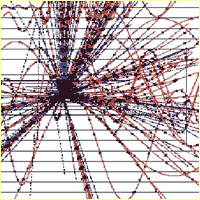This image is a simulation of the production and decay of a black hole in a proposed linear collider detector. The black hole quickly evaporates into every type of matter particle. The "democratic" selection of decay products is a distinct signature of black hole decay. Search for large extra dimensions The universe appears to be made of three dimensions of space and one of time. Objects go up and down, right and left, forward and backward, and move forward through time. This appearance, however, has never been tested at distances much less than the width of a hair, or at energies much above the mass of the top quark. What if there were additional directions in space in which particles could move if they just had enough energy? Einstein showed that the force of gravity was equivalent to the geometry of space and time. If there are more than three spacial dimensions, then the force of gravity is very different than normally experienced. The gravitational force between two particles is so weak compared to the other forces that it is generally ignored by particle physicists. In a world with additional dimensions, however, this gravity may be very strongly coupled to matter at high energies. This leads to the intriguing possibility of producing miniature black holes at collider experiments. These black holes would quickly decay by Hawking radiation into several particle/anti-particle pairs. Because gravitation couples to energy density, there is little preference for the type of particles that would appear in the decays. Hence, the signature of black hole production would be the appearance of nearly every type of particle coming from a point.Back to the Elegant Universe homepage for more articles, interviews, interactives, and slide shows.